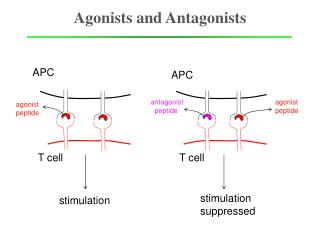DownloadDownload PresentationAgonists and Antagonists

# Agonists and Antagonists

Download Presentation## Agonists and Antagonists

- - - - - - - - - - - - - - - - - - - - - - - - - - - E N D - - - - - - - - - - - - - - - - - - - - - - - - - - -
##### Presentation Transcript

1. APC antagonist peptide agonist peptide T cell stimulation suppressed stimulation Agonists and Antagonists APC agonist peptide T cell

2. +ve -ve Minimal Model 3 species model: [X]+[Z]=M=const • Irreversibility • Branching • Feedback with distinct • time scale Minimal Model for cell signaling with positive and negative feedbacks

3. +ve -ve Minimal Model Mean field Analysis 3 species model: • Irreversibility • Branching • Feedback with distinct • time scale Rate equations : [X]+[Z]=M=const number conservation : initial values (t=0) : Minimal Model for cell signaling with positive and negative feedbacks

4. +ve -ve Minimal Model Mean field Analysis 3 species model: • Irreversibility • Branching • Feedback with distinct • time scale Solutions: [X]+[Z]=M=const stability: no un-stable mode Minimal Model for cell signaling with positive and negative feedbacks

5. +ve -ve Minimal Model Mean field Analysis 3 species model: • Irreversibility • Branching • Feedback with distinct • time scale [X]+[Z]=M=const Minimal Model for cell signaling with positive and negative feedbacks

6. Master Equation: Generating function: Solution: Effects of Stochastic Fluctuations 3 species model: Exactly Solvable

7. large N large k3 Fixed k3/N purely stochastic origin of bimodality Effects of Stochastic Fluctuations 3 species model: Exactly Solvable Stochastic Analysis Mean field Master Equation: small N small k3 Fixed k3/N [X]+[Z]=M=const Generating function: Solution:

8. X f ~ 1/(k2nxnynz) rate 1 P() Z rate 2 d ~ 1/(k3ny) Z d f Effects of Stochastic Fluctuations 3 species model: Exactly Solvable Origin of Stochastic Bistability (activation) Master Equation: [X]+[Z]=M=const (de-activation) Generating function: Solution:

9. agonist antagonist No signal Signaling product Cell Decision Mediated by Stochastic Fluctuations M. Artyomov, JD, M. Kardar, A. Chakraborty PNAS (2007)